# Edexcel Chemistry - Topic 9: Kinetics I

?
• Created by: Ryan C-S
• Created on: 06-04-18 19:48

## Collision Theory

• Reactions can only occur when collisions take place between particles which have sufficient energy
• The energy is usually needed to break the relevant bonds in the reactants
• The Activation Energy is defined as the minimum energy with which particles need to collide to start a reaction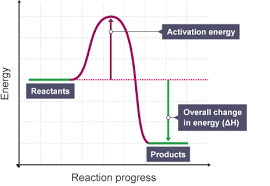1 of 7

## Maxwell Boltzmann Distribution

• The Maxwell-Boltzmann energy distribution shows the spread of energies that molecules of a gas or liquid have at a particular temperature
• The area under the curve represents the total number of particles present
• The Emp is the most probable energy that the particles will have
• The mean energy is the average amount of energy all the particles have
• A few particles have low energies because collisions cause some particles to slow down
• Only a few particles have energy greater than the Ea - these are the ones that can react
• The energy distribution should go through the origin as no molecules have no energy
• The energy distribution should never touch the x axis as there is no maximum energy for molecules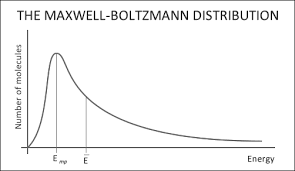2 of 7

## Increasing Temperatures and Surface Area

• As the temperature increases, the distribution shifts towards having more molecules with higher energies
• At higher temperatures both the Emp and the mean energy shift to higher energy values but the proportion of molecules with those energies decrease
• The total area underneath the curve should remain constant because the total number of particles is constant
• At higher temperatures the molecules have a wider range of energies
• A higher proportion of molecules have the activation energy
• Increasing Surface Area will cause successful collisions to occur more frequently between the reactant particles and this increases the rate of the reaction.3 of 7

## Increasing Concentrations and Pressures

• At higher concentrations and pressures, there are more particles per unit volume and so the particles collide with a greater frequency and there will be a higher frequency of effective collisions
• If concentration increases, the shape of the energy distribution curves don't change so the Emp and mean energy don't change
• The curves are higher and the area under the curves increases so there are more molecules with the Activation Energy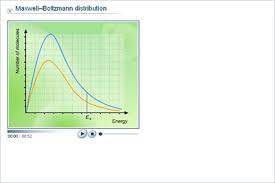4 of 7

## Measuring Reaction Rates

• The rate of reaction is the change in concentration of a substance per unit time. It is measured in mol dm-3s-1
• When a graph of concentration of reactant against time is plotted, the gradient of the curve is the rate of reaction
• The steeper the gradient the faster the rate of reaction
• When there is a plateau (flat section) on a graph, it means the reaction has finished.
• The initial rate of reaction is the rate at the start of the reaction where it is fastest
• Reaction rates can be calculated from graphs of reaction or product concentration; as the concentration of reactants decrease, the concentration of products increases at the same rate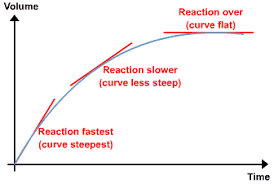5 of 7

## Effects of Catalysts

• A catalyst increases reaction rates without being used up by providing an alternative route or mechanism with a lower activation energy
• If the activation energy is lower, more particles will have an energy greater than the activation energy so there will be a higher frequency of effective collisions and so the reaction will be faster
• Catalysed reactions can occur at lower temperatures so less fuel is needed for a reaction so there are fewer emissions
• Catalysed reactions enables use of an alternative process with higher atom economy so meaning fewer materials are needed and less waste products are produced making a reaction more economically beneficial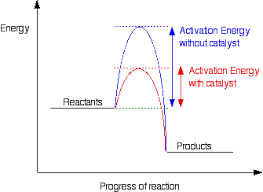6 of 7

## Heterogenous Catalysis

• A heterogenous catalyst is in a different phase from the reactants
• Heterogenous catalysts are usually solids whilst the reactants are gaseous or in solution. The reaction occurs on the surface of the catalyst
• The active site is the place where reactants adsorb onto the surface of the catalyst
• Adsorbtion of reactants at active sites on the surface may lead to catalytic action as the molecules are held in a more reactive configuration and there is a higher chance of the reactants being held on the surface being collided with sufficient energy to cause a reaction
• Increasing pressure has limited effect on the rate of heterogenous catalysed reactions because the reaction takes place on the surface of the catalyst and the active sites are already saturated with reactant molecules
• Catalysts speed up the rate of reaction but has no effect on equilibrium
7 of 7